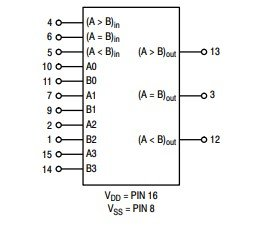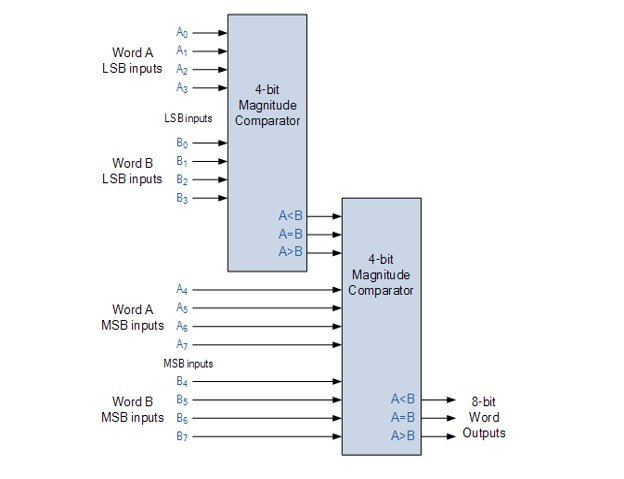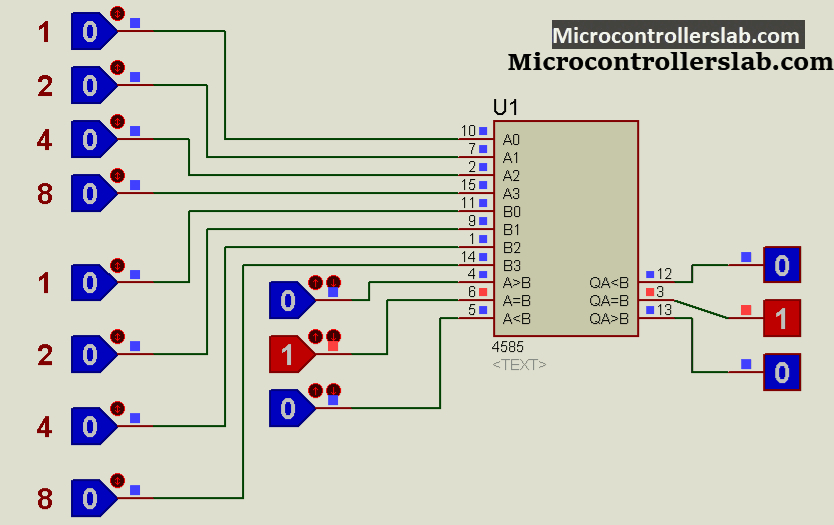# CD4585 4-Bit Magnitude Comparator IC

CD4585 is a magnitude comparator belonging to CMOS logic family. It is basically designed for applications that operates by the comparison of two quantities whether they are numbers or variables. Physical variables values can be converted into digital numbers through ADC converters to match their values against some reference or set point. The CD4585 compares two 4-bit BCD or binary numbers and determines whether the first 4-bit number is equal to, less than or greater than the second 4-bit number. This IC is best suitable for circuits that require lower power consumption.

## CD4585 Comparator Pinout Diagram

CD4585 Pinout diagram consists of sixteen pins. From out available pins three are output pins. Eleven pins peforms input functions including two 4-bit numbers and cascading pins.### Pin Configuration Details

As we mentioned earlier, tt has 16 GPIO pins total. All these pins along with their functions are described below:

Pin Name Description
A0-A3 4-bits of number A
B0-B3 4-bits of the number B
Pins 4, 5 and 6 Input pins used for cascading multiple units
(A=B) Out HIGH if magnitude of 1st digit is equal to B0-B3 otherwise this pin is LOW
(A>B) Out Output high, if magnitude of 1st digit is greater than B0-B3 otherwise otherwise this pin is LOW
(A<B) Out HIGH when first number is less than second number otherwise this pin is LOW
VDD Positive Supply
Vss Negative Supply

## CD4585 Features

• It can compare 4N bit numbers by cascading multiple ICs.
• It performs its operation at medium speed by comparing numbers in typically 180ns at 10V.
• 100% tested for quiescent current at 20 V
• It has a symmetrical output.
• Parametric ratings: 5V, 10V and 15V
• At 18V, the maximum input current is:
• 1 µA over full package temperature range
• 100 nA at 25°C
• The value of noise margin over full package temperature range for supply voltages 5V, 10V and 15V is 1V, 2V and 2.5V
• It is applicable for both Binary and BCD Code
• It has a wide Voltage Supply Range of 3 to 18 V.
• CD4585 has a capability of driving two TTL Loads of low power.

## How to Use it CD4585 Comparator

In this section, we will see how this 4-bit comparator IC works. Let’s start with a comparison of 4-bit number. Although we can comparate 4N bit number. Firstly, for the comparison of two 4-bit numbers, the connections of cascading inputs are as follows:

• Pin4= LOW
• Pin5 = HIGH
• Pin6 = HIGHYou can also compare numbers with more than 4 bits by cascading multiple devices that would expand the comparator function to 4N bits. For cascading, the cascading inputs of the least significant IC are connected to the cascading inputs of the most significant IC. The pins 4, 5, 6 of the most significant comparator are connected in the same way as in the comparison of 4-bit numbers. Suppose you want to design an 8-bit magnitude comparator. For this, you’ll have to cascade two comparators by connecting their cascading inputs in the sequence mentioned above. You can see from the diagram how to connect the cascading inputs.### Proteus Simulation

In this example, we use loggle switches to demonstrate the working of CD4585 comparator. Because we are using it for 4-bit comparison only. Therefore, we connect cascading pins such A>B, A=B, A<B with logicS 010 respectively. Numbers besides every toggle switch shows the magnitude of each binary bit in decimal.

Proteus has a built in library of CD4585 comparator. Hence, we can easliy simulate this IC.### Alternative Options

Other comparators are CD4063, CD4585B

## CD4585 Applications

This IC is used in many circuits including:

• Null Detection Circuits
• Level Shifter
• Analogue to Digital Converters
• Central Processing Unit of Computers

### 1 thought on “CD4585 4-Bit Magnitude Comparator IC”

1. Please help me how did you made this simulation, i need it for my project cause i want to build it, Thankyou